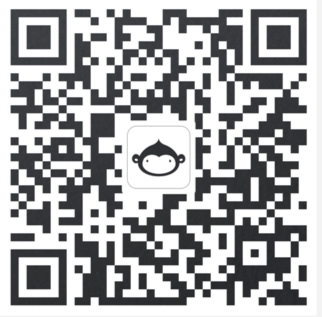# 编辑器 如何显示图片的问题？

## 样例代码 python

``````import matplotlib.pyplot as plt
x = [1,2,3]
y = [10,5,7]
plt.plot(x,y)
plt.show()
print("show me a picture")
``````

## 终端执行结果

@aiml 下午话，很荣幸为您服务。 CloudStudio 没有 GUI 界面，您可参考下面代码，直接生成一个图片进行浏览。如您还有其他问题，您可以直接添加我们下方专属客服的微信，我们会安排相关的客服经理尽快联系到您解答您的问题，再次感谢您的咨询。

``````import matplotlib as mpl

mpl.use('Agg')

import matplotlib.pyplot as plt

x = [1, 2, 3, 4]

y = [1.2, 2.5, 4.5, 7.3]

plt.plot(x, y)

plt.show()

plt.savefig('a.png')
``````